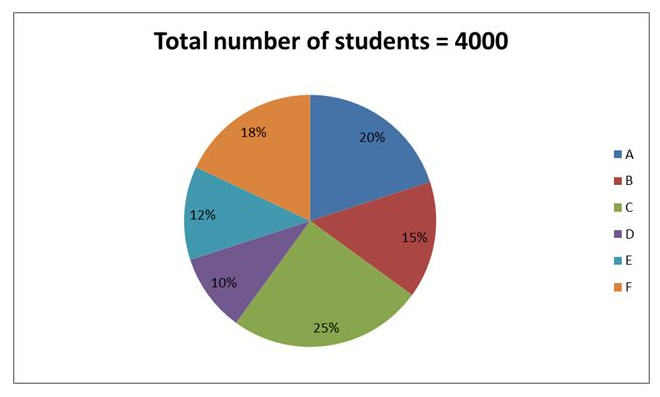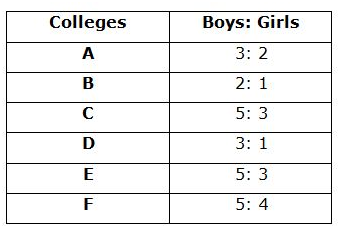# IBPS PO Prelims Quantitative Aptitude (Day-06)

Dear Aspirants, Our IBPS Guide team is providing new series of Quantitative Aptitude Questions for IBPS PO Prelims 2020 so the aspirants can practice it on a daily basis. These questions are framed by our skilled experts after understanding your needs thoroughly. Aspirants can practice these new series questions daily to familiarize with the exact exam pattern and make your preparation effective.

Start Quiz

Approximation

Direction (1-5): What approximate value should come in the place of (?) in the following questions?

1) 190.12 * 7.921 – 77.771 % of 630.12 – 9.0909 % of 550.12 = ?

A) 980

B) 880

C) 860

D) 840

E) 820

2) 105.12 % of 239.920 + ? % of 23.12 = 413.912

A) 700

B) 900

C) 800

D) 600

E) 400

3) 33.12 * √7055 – 164.512 * 16.02 = ?

A) 100

B) 150

C) 120

D) 130

E) 140

4) 783.12 ÷ 9.12+ 99.908 + 11.11 % of 26.901 = ?

A) 120

B) 140

C) 160

D) 190

E) 180

5) 15.123 % of 120.123 – 13.13 * 16.910 + 79.920 = ?

A) -123

B) -148

C) -133

D) -153

E) -167

Data interpretation

Direction (6-10): Study the following information carefully and answer the questions given below.

The given pie chart shows the number of students from six different colleges.The given table shows the ratio of boys to girls in six different colleges.6) What is the ratio of the number of girls from A to the number of girls from E?

A) 16: 9

B) 13: 11

C) 17: 8

D) 19: 10

E) None of these

7) What is the average number of boys from all the colleges together excluding F?

A) 421

B) 423

C) 425

D) 438

E) None of these

8) The number of girls from C is approximately what percent of the total number of students from F?

A) 48%

B) 52%

C) 56%

D) 60%

E) None of these

9) What is the difference between the number of girls from B and C together and the number of girls from E and F together?

A) 65

B) 70

C) 75

D) 80

E) None of these

10) What is the difference between the average number of boys from A, D and E together and the average number of students from B, E and F together?

A) 180

B) 200

C) 220

D) 240

E) None of these

Directions (1-5):

190.12 * 7.921 – 77.771 % of 630.12 – 9.0909 % of 550.12 = ?

1520 – 490 – 50 = ?

? = 980

105.12 % of 239.920 + ? % of 23.12 = 413.912

252 + ? % 23 = 414

? % 23 = 162

? = 700

33.12 * √7055 – 164.512 * 16.02 = ?

2772 – 2632 = ?

? = 140

783.12 ÷ 9.12+ 99.908 + 11.11 % of 26.901 = ?

87 + 100 + 3 = ?

190 = ?

15.123 % of 120.123 – 13.13 * 16.910 + 79.920 = ?

18 – 221 + 80 = ?

-123 = ?

Directions (6-10):Required ratio = 320: 180

= 16: 9

Required Average = (480 + 400 + 625 + 300 + 300)/5

= 421

Required percentage = 375/720 * 100 = 52%

Number of girls from B and C = 200 + 375 = 575

Number of girls from E and F = 180 + 320 = 500

Difference = 575 – 500 = 75

Average boys from A, D and E = 480 + 300 + 300/3 = 360

Average students from B, E and F = 600 + 480 + 720/3 = 600

Difference = 600 – 360 = 240

 Check Here to View IBPS PO Prelims 2020 Quantitative Aptitude Questions Day – 05 Day – 04 Day – 03
1 1 vote
Rating